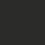# Number Theory: Divisibility

This is the first note in the series Number Theory: Divisibility. All numbers involved in this note are integers, and letters used in this note stand for integers without further specification.

Numbers involved in this note are integers, and letters used in this book stand for integers without further specification.

Given numbers $a$ and $b$, with $b \neq 0$, if there is an integer $c$, such that $a=bc$, then we say $b$ divides $a$, and write $b \mid a$. In this case we also say $b$ is a factor of $a$, or $a$ is a multiple of $b$. We use the notation $b \nmid a$ when $b$ does not divide $a$ (i.e., no such $c$ exists).

Several simple properties of divisibility could be obtained by the definition of divisibility (proofs of the properties are left to readers).

$(1)$ If $b \mid c,$ and $c \mid a,$ then $b \mid a,$ that is, divisibility is transitive.

$(2)$ If $b \mid a,$ and $b \mid c,$ then $b \mid (a \pm c),$ that is, the set of multiples of an integer is closed under addition and subtraction operations.

By using this property repeatedly, we have, if $b \mid a$ and $b \mid c$, then $b \mid (au+cv)$, for any integers $u$ and $v$. In general, if $a_1,a_2,\cdots,a_n$ are multiples of $b$, then $b \mid (a_1+a_2+\cdots+a_n).$

$(3)$ If $b \mid a$, then $a=0$ or $\lvert a \rvert \geq \lvert b \rvert$. Thus, if $b \mid a$ and $a \mid b$, then $\lvert a \rvert = \lvert b \rvert$.

Clearly, for any two integers $a$ and $b$, $a$ is not always divisible by $b$. But we have the following result, which is called the division algorithm. It is the most important result in elementary number theory.

$(4)$ (The division algorithm) Let $a$ and $b$ be integers, and $b > 0$. Then there is a unique pair of integers $q$ and $r$, such that $a=bq+r \hspace{2mm} \text{and} \hspace{2mm} 0 \leq r < b.$

The integer $q$ is called the (incomplete) quotient when $a$ is divided by $b$, $r$ called the remainder. Note that the values of $r$ has $b$ kinds of possibilities, $0,1,\cdots,b-1$. If $r=0$, then $a$ is divisible by $b$.

It is easy to see that the quotient $q$ in the division algorithm is in fact $\lfloor\frac{a}{b}\rfloor$ (the greatest integer not exceeding $\frac{a}{b}$), and the heart of the division algorithm is the inequality about the remainder $r$: $0 \leq r < b$. We will go back to this point later on.

The basic method of proving $b \mid a$ is to factorize $a$ into the product of $b$ and another integer. Usually, in some basic problems this kind of factorization can be obtained by taking some special value in algebraic factorization equations. The following two factorization formulae are very useful in proving this kind of problems.

$(5)$ If $n$ is a positive integer, then $x^n-y^n=(x-y)(x^{n-1}+x^{n-2}y+\cdots+xy^{n-2}+y^{n-1}).$

$(6)$ If $n$ is a positive odd number, then $x^n+y^n=(x+y)(x^{n-1}-x^{n-2}y+\cdots-xy^{n-2}+y^{n-1}).$Note by Victor Loh
5 years, 9 months ago

This discussion board is a place to discuss our Daily Challenges and the math and science related to those challenges. Explanations are more than just a solution — they should explain the steps and thinking strategies that you used to obtain the solution. Comments should further the discussion of math and science.

When posting on Brilliant:

• Use the emojis to react to an explanation, whether you're congratulating a job well done , or just really confused .
• Ask specific questions about the challenge or the steps in somebody's explanation. Well-posed questions can add a lot to the discussion, but posting "I don't understand!" doesn't help anyone.
• Try to contribute something new to the discussion, whether it is an extension, generalization or other idea related to the challenge.

MarkdownAppears as
*italics* or _italics_ italics
**bold** or __bold__ bold
- bulleted- list
• bulleted
• list
1. numbered2. list
1. numbered
2. list
Note: you must add a full line of space before and after lists for them to show up correctly
paragraph 1paragraph 2

paragraph 1

paragraph 2

[example link](https://brilliant.org)example link
> This is a quote
This is a quote
    # I indented these lines
# 4 spaces, and now they show
# up as a code block.

print "hello world"
# I indented these lines
# 4 spaces, and now they show
# up as a code block.

print "hello world"
MathAppears as
Remember to wrap math in $$ ... $$ or $ ... $ to ensure proper formatting.
2 \times 3 $2 \times 3$
2^{34} $2^{34}$
a_{i-1} $a_{i-1}$
\frac{2}{3} $\frac{2}{3}$
\sqrt{2} $\sqrt{2}$
\sum_{i=1}^3 $\sum_{i=1}^3$
\sin \theta $\sin \theta$
\boxed{123} $\boxed{123}$

Sort by:

Thanks much for posting this. I would love if you could name the book you had referred to in the beginning of this note!

- 5 years, 9 months ago

I'm not sure if I want to reveal it because I'm scared I'm taking a little too much information :D

- 5 years, 9 months ago

I like the fact that although it's meant to be a comment with a negative connotation, there's still a smiley icon at the back which doesn't really fit the sentence (lol). Also, I think I have an idea which book he may have referred to...

- 5 years, 9 months ago

But still, we have to acknowledge the rules of copyright. IF I feel like checking this book and find that you have really gleaned too much information from it, I might have to report you... D:

- 5 years, 9 months ago

It's not THAT book, I promise.

- 5 years, 9 months ago

Anyway, Brilliant is a site for sharing information, and I haven't exactly shared the examples and exercises yet.

- 5 years, 9 months ago

Uh-Oh!

- 5 years, 9 months ago